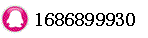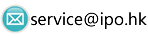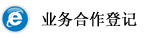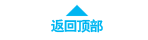# Terminal Value - TV时间：2018-05-02 17:33:09     浏览：100    评论：0

The value of an investment at the end of a period, taking into account a specified rate of interest.

|||The formula to calculate terminal is the same as that for compound interest:

Where:
TV = the total amount
P = the principal amount
r = interest rate
t = period of time

0相关评论联系我们